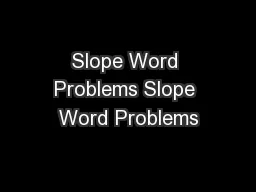# Slope Word Problems Slope Word ProblemsEmbed code:

## Slope Word Problems Slope Word Problems

Download Presentation - The PPT/PDF document "Slope Word Problems Slope Word Problems" is the property of its rightful owner. Permission is granted to download and print the materials on this web site for personal, non-commercial use only, and to display it on your personal computer provided you do not modify the materials and that you retain all copyright notices contained in the materials. By downloading content from our website, you accept the terms of this agreement.

### Presentations text content in Slope Word Problems Slope Word Problems

Slide1

Slope Word Problems

Slide2

Slope Word Problems

Lesson Objective: 4.01a

Students will know how to solve word problems using slope

Slide3

Slope Word Problems

In 2005, Joe planted a tree that was 3 feet tall. In 2010, the tree was 13 feet tall. Assuming the growth of the tree is linear

,

what was the rate of growth of the tree?

Slide4

Slope Word Problems

In 2005, Joe planted a tree that was 3 feet tall. In 2010, the tree was 13 feet tall. Assuming the growth of the tree is linear, what was the rate of growth of the tree?

What does “rate of growth” mean?

Slope!

Slide5

Slope Word Problems

Remember the slope equation:

In order to find the slope we need two points

m =

y

2

– y

1

x

2

– x

1

Slide6

Slope Word Problems

In 2005, Joe planted a tree that was 3 feet tall. In 2010, the tree was 13 feet tall. Assuming the growth of the tree is linear, what was the rate of growth of the tree?

Remember, x is always years, so replace x

2

with the second year and x

1

with the first

y

2

– y

1

m =

x

2 – x1

2010 – 2005

Slide7

Slope Word Problems

In 2005, Joe planted a tree that was 3 feet tall. In 2010, the tree was 13 feet tall. Assuming the growth of the tree is linear, what was the rate of growth of the tree?

Replace

y

2

with the height from the second year and y

1

with the first

y

2

– y

1

m =

2010 – 200513 – 3

Slide8

Slope Word Problems

Simplify the top

Simplify the bottom

Simplify the fraction to get m. Keep it as a fraction if it can’t be simplified

m =

2010 – 2005

13 – 3

10

5

2

Slide9

Slope Word Problems

In 2005, Joe planted a tree that was 3 feet tall. In 2010, the tree was 13 feet tall. Assuming the growth of the tree is linear, what was the rate of growth of the tree?

The slope is 2, so the tree grows 2 feet per year

m = 2

Slide10

Slope Word Problems

In 1995 a public library had 16,000 books on its shelves. In 1999 the library had 19,000 books. Assuming a linear increase, how many books were added to the library each year?

Slide11

Slope Word Problems

In 1995 a public library had 16,000 books on its shelves. In 1999 the library had 19,000 books. Assuming a linear increase, how many books were added to the library each year?

We’re looking for how many for each year, usually the word “each” means slope.

Slide12

Slope Word Problems

In 1995 a public library had 16,000 books on its shelves. In 1999 the library had 19,000 books. Assuming a linear increase, how many books were added to the library each year?

Years are always x so replace the

x’s

with the years

Replace the

y’s

with the number of books for each year

(x

1

, y

1

), (x2

, y2)(1995,

y

1

),

(1999,

y

2

)

(1995, 16000), (1999, 19000)

Slide13

Slope Word Problems

Replace y

2

with 19000 and y

1

with 16000

Replace

x

2

with

1999 and x1 with 1995Simplify the top and the bottomReduce the fraction

(1995, 16000), (1999, 19000)m =y

2 – y1x

2

x

1

19000 – 16000

1999 – 1995

3000

4

750

Slide14

Slope Word Problems

In 1995 a public library had 16,000 books on its shelves. In 1999 the library had 19,000 books. Assuming a linear increase, how many books were added to the library each year?

m = 750, therefore the library adds 750 books each year

m = 750

Slide15

Slope Word Problems

Monica feeds

her

dog the same amount of dog food each day from a very large bag. ON the 3

rd

day, she has 44 cups left in the bag, and on the 11

th

day she has 28 cups left. How many cups of food does she feed her dog a day?

Slide16

Slope Word Problems

Wendy bought a car for \$25,000 and its value depreciated linearly. After 3 years the value was \$21,250. What was the amount of yearly depreciation?

Slide17

Slope Word Problems

Jamal’s parents give him \$20 to spend at camp. Jamal spends the same amount of money on snacks each day. After 4 days he has \$12 left. How much money is he spending

each day?

Slide18

Slide19